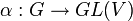# Continuous linear representation of compact group over reals has invariant symmetric positive-definite bilinear form

## Statement

### For finite-dimensional vector space

Suppose$G$ is a compact group and$\alpha:G \to GL(V)$ (with$V$ finite-dimensional) is a linear representation of$G$ over a vector space$V$ over$\R$, the field of real numbers. Then, there exists a symmetric positive-definite bilinear form$b: V \times V \to V$ such that$\! b(\alpha(g)v,\alpha(g)w) = b(v,w) \ \forall \ g \in G, \ v,w \in V$

In other words, we can think of$b$ as a dot product invariant under the action of$G$.

Further, if we choose a basis for$V$ that is an orthonormal basis for$b$, then in this basis, all the matrices for$\alpha(g), g \in G$, are orthogonal matrices. Thus, another formulation is that every continuous finite-dimensional linear representation over the real numbers is an orthogonalizable linear representation.